The Chi-Square Distribution

# 56 Facts About the Chi-Square Distribution

The notation for the chi-square distribution is: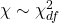where df = degrees of freedom which depends on how chi-square is being used. (If you want to practice calculating chi-square probabilities then use df = n – 1. The degrees of freedom for the three major uses are each calculated differently.)

For the χ2 distribution, the population mean is μ = df and the population standard deviation is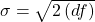.

The random variable is shown as χ2.

The random variable for a chi-square distribution with k degrees of freedom is the sum of k independent, squared standard normal variables.

χ2 = (Z1)2 + (Z2)2 + … + (Zk)2

1. The curve is nonsymmetrical and skewed to the right.
2. There is a different chi-square curve for each df.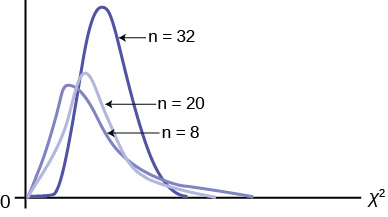3. The test statistic for any test is always greater than or equal to zero.
4. When df > 90, the chi-square curve approximates the normal distribution. For X ~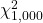the mean, μ = df = 1,000 and the standard deviation, σ == 44.7. Therefore, X ~ N(1,000, 44.7), approximately.
5. The mean, μ, is located just to the right of the peak.

### References

“HIV/AIDS Epidemiology Santa Clara County.”Santa Clara County Public Health Department, May 2011.

### Chapter Review

The chi-square distribution is a useful tool for assessment in a series of problem categories. These problem categories include primarily (i) whether a data set fits a particular distribution, (ii) whether the distributions of two populations are the same, (iii) whether two events might be independent, and (iv) whether there is a different variability than expected within a population.

An important parameter in a chi-square distribution is the degrees of freedom df in a given problem. The random variable in the chi-square distribution is the sum of squares of df standard normal variables, which must be independent. The key characteristics of the chi-square distribution also depend directly on the degrees of freedom.

The chi-square distribution curve is skewed to the right, and its shape depends on the degrees of freedom df. For df > 90, the curve approximates the normal distribution. Test statistics based on the chi-square distribution are always greater than or equal to zero. Such application tests are almost always right-tailed tests.

### Formula Review

χ2 = (Z1)2 + (Z2)2 + … (Zdf)2 chi-square distribution random variable

μχ2 = df chi-square distribution population mean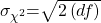Chi-Square distribution population standard deviation

If the number of degrees of freedom for a chi-square distribution is 25, what is the population mean and standard deviation?

mean = 25 and standard deviation = 7.0711

If df > 90, the distribution is _____________. If df = 15, the distribution is ________________.

When does the chi-square curve approximate a normal distribution?

when the number of degrees of freedom is greater than 90

Where is μ located on a chi-square curve?

Is it more likely the df is 90, 20, or two in the graph?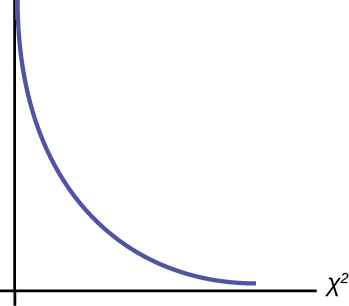df = 2

### Homework

Decide whether the following statements are true or false.

As the number of degrees of freedom increases, the graph of the chi-square distribution looks more and more symmetrical.

true

The standard deviation of the chi-square distribution is twice the mean.

The mean and the median of the chi-square distribution are the same if df = 24.

false